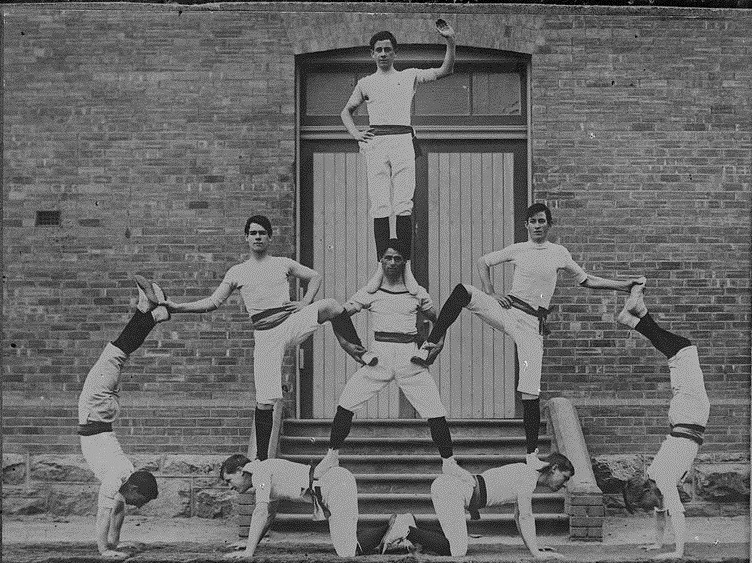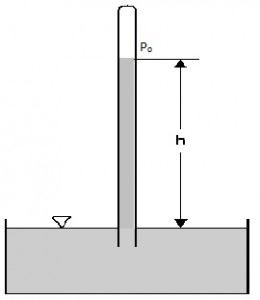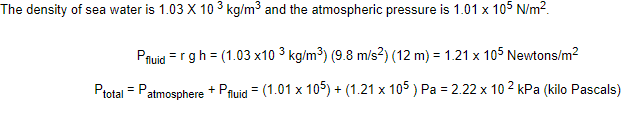# Fluid Pressure - Hydrostatic PressureIn a human pyramid, the person in the bottom-most row carries the most load than any other person in the pyramid.

Have you ever seen a human pyramid? Imagine the condition of the guy in the bottom-most row. He is obviously carrying a lot more load than the guy on the 1st row. The same is the case with fluid pressure in a column. In a fluid column, as the depth increases, the pressure increases as well. And why does this pressure increase? Because as you go deeper, fluid at a lower depth has to support fluid above it as well. Therefore to define it, we can say that

Fluid pressure is the pressure at a point within a fluid arising due to the weight of the fluid.

The pressure in fluids can be calculated using the following relation.Pfluid = P + ρgh

where,

P = Pressure at the reference point

Pfluid is the pressure at a point in a fluid

ρ is the density of the fluid

g is the acceleration due to gravity (considering earth g = 9.8 m/s)

h is the height from the reference point

The density of the fluid can be calculated by dividing the mass of the fluid in consideration with the volume of fluid taken.

ρ = m/v

where,

m is the mass of the fluid

v is the volume of fluid considered

If the fluid is subject to atmospheric pressure than the total pressure on the system is given by

Pfluid = Po + ρgh

where,

Po is the atmospheric pressure

If you prefer a video explanation of fluid pressure, then watch the video given below.## Conditions for the Consideration of Fluid Pressure:

Fluid pressure is considered in two conditions:

1. In an open condition or open channel flow
2. In a closed condition or closed conduit

The fluid pressure given above is the static fluid pressure or hydrostatic pressure. The pressure here is considered based only on the depth of fluid because the pressure produced due to fluid movement can be considered negligible. The static fluid pressure does not depend on the surface area, the shape of the fluid container or total volume and mass of the liquid.

Keep in mind that here, fluids refer to anything that has the ability to flow. Therefore, this refers to both liquids and gases.

## Solved Example

1. Calculate the pressure exerted on a scuba driver when she is 12 meters below the surface of the ocean. Assume the standard atmospheric condition.
Solution:### Factors That Affect Fluid Pressure

There are two factors that affect fluid pressure. These two factors are the depth of the fluid and its density.

• Depth of the fluid: As the depth increases, the pressure exerted by the fluid becomes more.
• Density of the fluid: Denser fluids such as water exert more pressure than lighter fluids such as air. The molecules in the denser fluid are close to each other resulting in more collisions in the given area. This results in the exertion of more pressure.

## Frequently Asked Questions – FAQs

### How to calculate fluid pressure?

There are three methods that can be used for the calculation of fluid pressure. Also, fluid pressure is dependent on the density and height of the fluid and is independent of the mass of the fluid.

• By calculating the fluid pressure
• By finding the density of the liquid
• By considering the variables in the equation of fluid pressure

### Why do fluids exert pressure?

The fluid exerts pressure in all directions because the particles in the fluid can move in all directions. The movement of these molecules results in continuous collisions. The collision causes pressure which is exerted in all directions.

### What causes fluid to flow?

There are three factors that cause fluid to flow:

• The characteristics of fluid such as viscosity, density, and compressibility
• The speed of the flow
• The shape of the surface

### What will increase gas pressure?

Gas pressure is dependent on the temperature, pressure, number of molecules, and the volume.

• When the number of molecules in the gas increases, there is an increase in gas pressure.
• When the volume decreases, the gas pressure increases.
• As the temperature increases, the gas pressure increases.

### How does temperature affect gas pressure?

We know that the temperature of the gas is proportional to the average kinetic energy of the molecules. As the kinetic energy increases, the collision between the molecules increases resulting in increased pressure in the gas.

Test Your Knowledge On Fluid Pressure!